Primary maths: Addition and subtraction

Resources team
16th September 2019Conquer your pupils' anxiety about addition and struggles with subtraction using these tried-and-tested classroom resources

Whether your students are using their fingers, counters or mental arithmetic to add and subtract, taking the time to cement their understanding is essential at this time of year. To help your class grasp the basics, we've done the hard work for you and found some of the very best addition and subtraction resources that the Tes community have to offer. So, what are you waiting for?Y1 Addition and subtraction worksheets

Made for Year 1 with 3 way differentiation. Simple number lines included.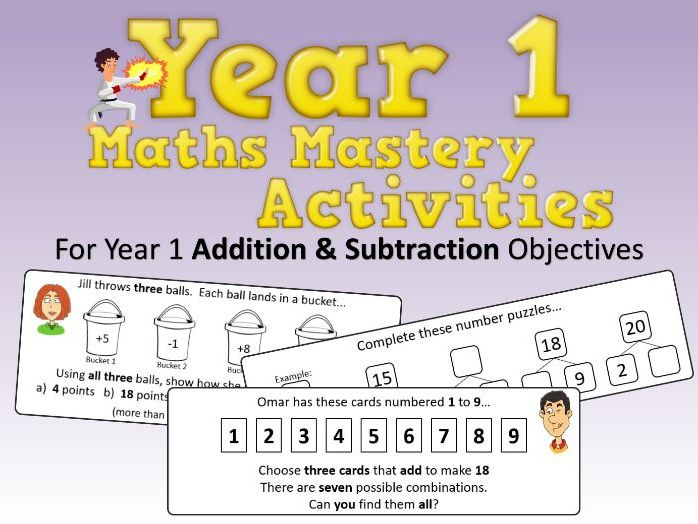Addition and Subtraction Mastery Activities – Year 1

Click the link below for the complete Year 1 resource pack (all 21 objectives)

Year 1 Maths Mastery Pack

These are a range of activities/tasks to demonstrate mastery and develop deeper thinking and deeper understanding of year 1 Addition and Subtraction objectives. For each objective there are at least 3 activities/tasks along with answers (where possible). The document is set up so that you can quickly jump to the objective you are covering and then simply print the appropriate pages. In addition, almost all the activities can be adapted to suit your class’s needs if necessary.

Finding or creating resources to evidence mastery and deeper understanding can take hours of time (and a lot of frustration). With this resource, you will have a handy bank of activities/tasks that you can choose from in seconds!

Objectives covered:

Read, write and interpret mathematical statements involving addition (+), subtraction (−) and equals (=) signs
Represent and use number bonds and related subtraction facts within 20
Add and subtract one-digit and two-digit numbers to 20, including 0
Solve one-step problems that involve addition and subtraction, using concrete objects and pictorial representations, and missing number problems such as 7 = ? – 9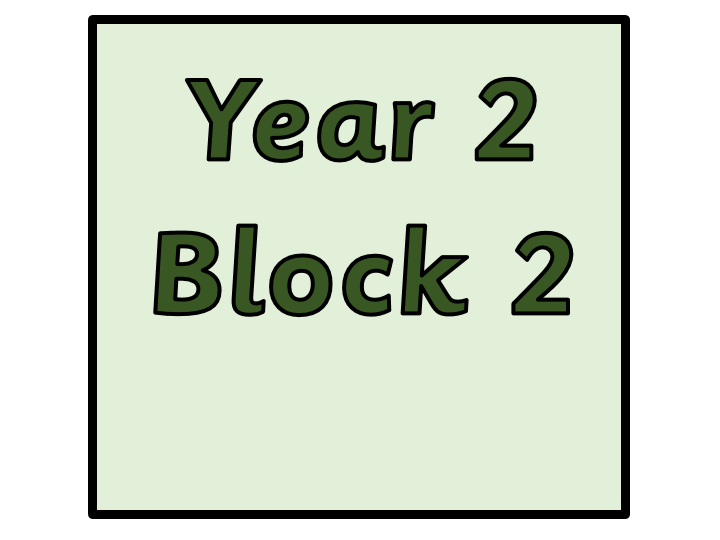Year 2 - Block 2 Addition and Subtraction

I have created 50 worksheets linked to the Small Steps Guidance for White Rose Maths Block 2: Addition and Subtraction.

All the worksheets fit with the small steps. I will be using these when I deliver to my Year 2 class.

I have produced them all in Sassoon Primary styled font.

I have other resources including IAF linked maths document for PKS< Working Towards and Working at STandard. Also, I have 5 practice SATs Arithmetic papers which have been created in the same layout as the real thing.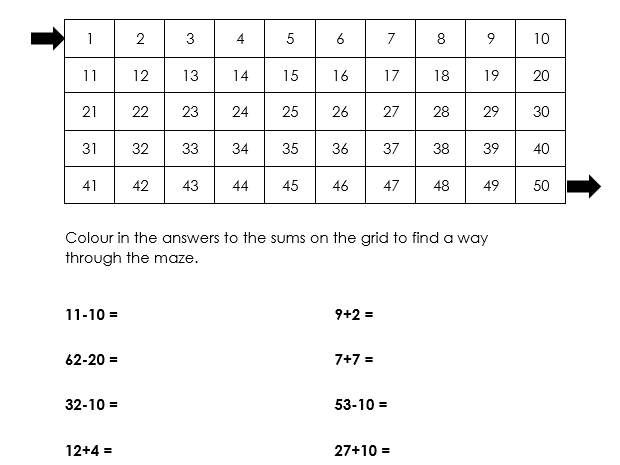Year 2 addition and subtraction maze problem

Year 2 Maths objective: add and subtract a two-digit number and ones/a two-digit number and tens. A fun activity to practise these objectives to solve a simple problem. I also used this with my lower-ability Year 3s. Could be adapted for different year groups and also for multiplication/division. Could also be used as a whole-class activity as a starter or plenary.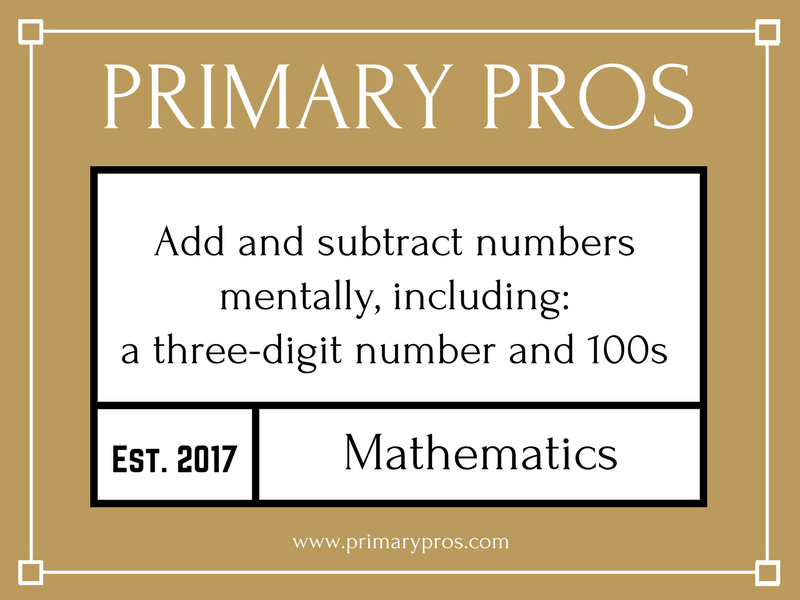Add and subtract numbers mentally, including: a three-digit number and 100s

A detailed and engaging PowerPoint, Differentiated (SEN, LA, MA, HA & Mastery) Worksheet with Answer Sheet for a complete lesson on this National Curriculum Statement: Add and subtract numbers mentally, including: a three-digit number and 100s.

The PowerPoint has the following features:

→ Differentiated starter activity
→ Extremely logical and simplistic explanations with animated examples and pictures to aid learning
→ Learning Objective and differentiated success criteria
→ Animated count-down timer so children know how long they have left of the lesson

The Word Document has the following features:

→ Differentiated activities on one sheet from SEN, LA, MA, HA and Mastery Challenge
→ Coloured activity boxes to help you quickly assign tasks to children
→ Learning objective and short date (which automatically updates to the day you open the document)
→ Differentiated success criteria that link with the PowerPoint
→ Answer sheet included for all questions

Explore our other Year 3 Addition & Subtraction Lessons: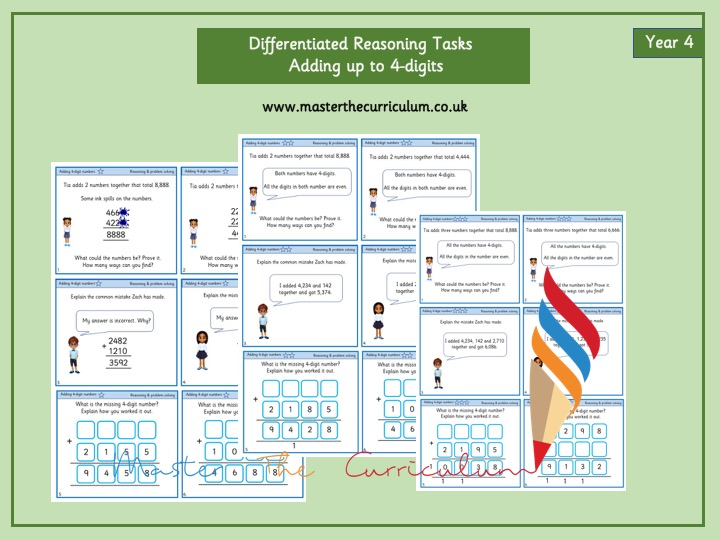Year 4- Differentiated Reasoning Tasks- Adding 4-digit numbersAddition and Subtraction Word Problems

Year 5 Addition and Subtraction 1 and 2 step word problems with 4 or more digit numbers.
Two documents - one core, one more able.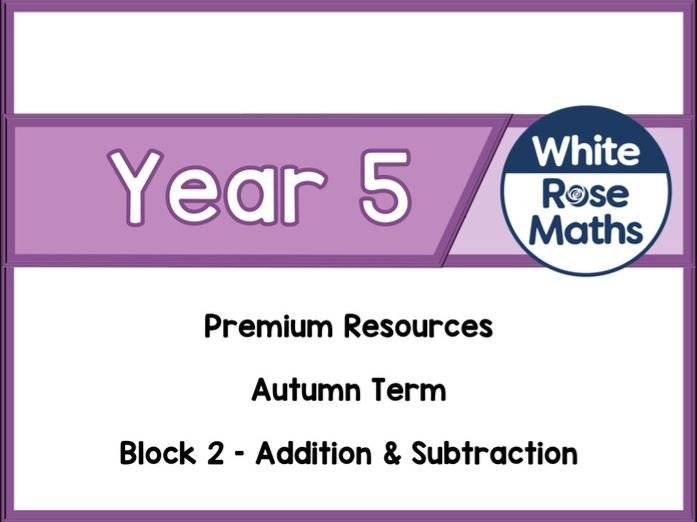Year 5 - Addition & Subtraction

White Rose Maths is pleased to announce its premium purposeful practice resources. These resources are available for one off purchase through the TES or as part of individual and school subscription packages on our own site https://resources.whiterosemaths.com
These one off purchases are aimed at schools who just want particular one off resources to teach particular areas of the curriculum.

These resources are for Year 5 – Addition & Subtraction - 5 small steps

Included is the following for each step of the White Rose Maths curriculum

A 2 page write on purposeful practice worksheet
A display version of the same worksheet. This also can be used as a non-write on version.
PowerPoint which contains each of the questions from the worksheets, one slide per question. Ideal for whole class display and discussion.
All the answers to all the problems.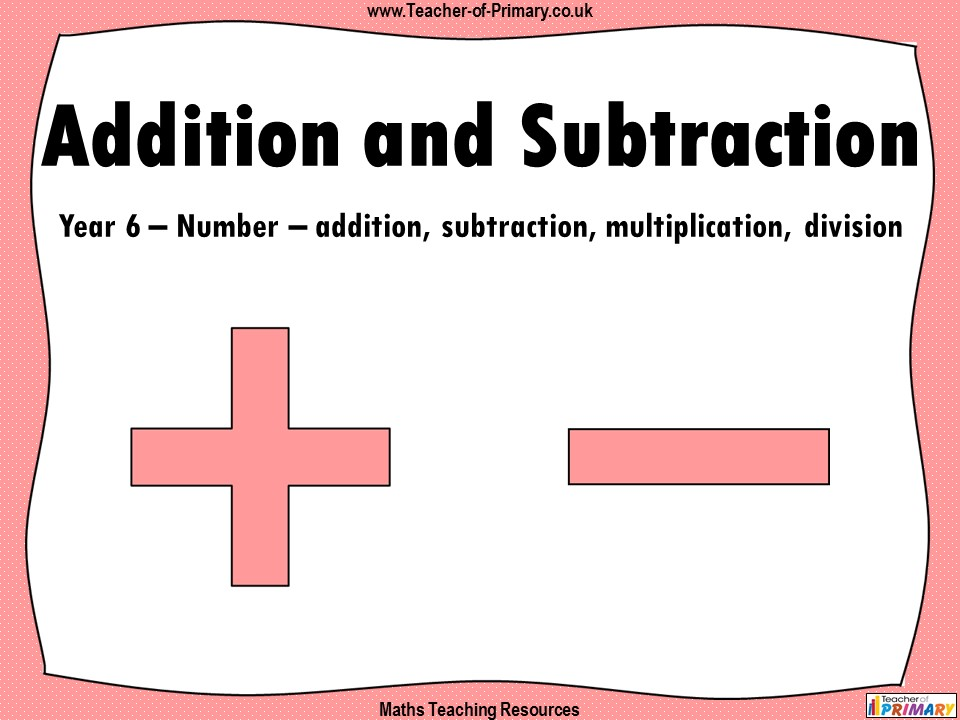Addition and Subtraction - Year 6

Addition and Subtraction - Year 6

This Year 6 maths teaching resource includes a PowerPoint and differentiated worksheets for independent learning, to guide your class through using addition and subtraction to solve calculations and multi-step problems as per the curriculum objectives of the Year 6 maths programme of study (Number - addition, subtraction, multiplication and division). It will help the children understand how to solve addition and subtraction problems in context and the resource helps children learn which operation to use and why. The worksheets contain varied fluency, reasoning and problem solving to reinforce and consolidate the children’s learning. The resource contains two lessons, which support the White Rose Small Steps guidance, and are designed to support a mastery approach to teaching maths.

Content includes:

• Lessons 1 and 2: Addition and Subtraction with 2 accompanying worksheets with answers

‘Addition and Subtraction - Year 6’ is completely editable giving teachers the freedom to adapt the resource to suit their individual teaching needs.

‘Addition and Subtraction - Year 6’ is included in our complete teaching unit ‘The Four Operations - Year 6’. Click on the link below to take a closer look.

https://www.tes.com/teaching-resource/the-four-operations-year-6-12157420Missing numbers column addition and subtraction (Year 6)

One page of 5 digit column addition and column subtraction problems with missing numbers.
I use these in year 6 - probably good for year 5 too!
Good variation on adding and taking away.
Very similar to the SATs questions too.